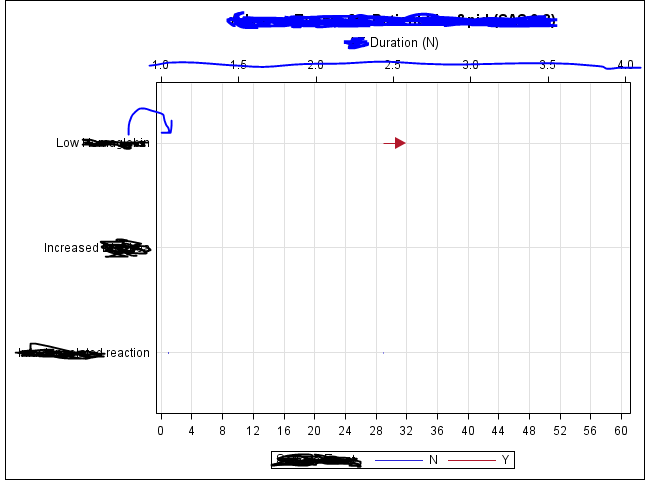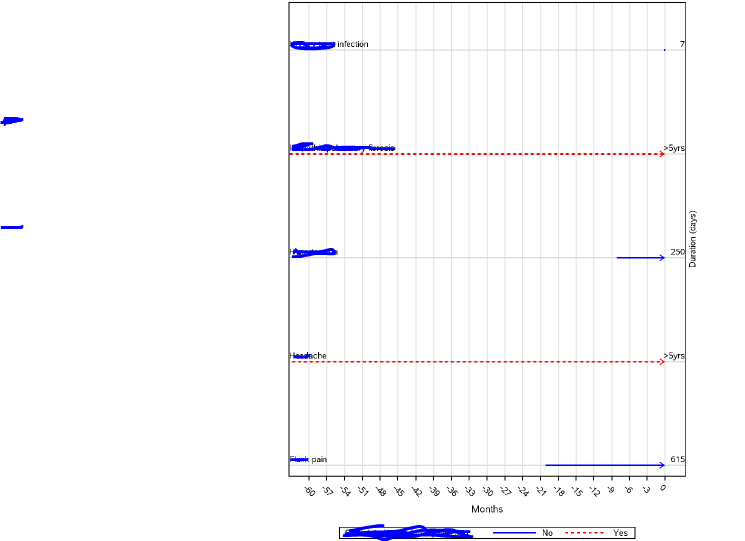## Doubt on SAS graph

Hi all,

I am getting 1 st diagram as my output but I needed output same as like the 2nd diagram.

I have used the following code.

proc sgplot data=graph;

highlow y=term low=stdy high=endy / type=line group=ser
lineattrs=(pattern=solid) barwidth=0.8 highcap=FILLEDARROW ;
scatter y=term x=adurn / x2axis markerattrs=(size=0);
xaxis grid display=(nolabel) offsetmax=0.02 values=(0 to 60 by 2);
yaxis grid display=(noticks nolabel);
run;

1. the Plot term should present inside the graph. (I have specified with arrow)

2. I have to specify condition at the end plot. (please refer to the second diagram)

Thanks in advance.3 REPLIES 3

## Re: Doubt on SAS graph

Data.

Hard to plot something we don't have.

How do we know what is supposed to be plotted with the dashed line?

Instructions here: https://communities.sas.com/t5/SAS-Communities-Library/How-to-create-a-data-step-version-of-your-dat... will show how to turn an existing SAS data set into data step code that can be pasted into a forum code box using the {i} icon or attached as text to show exactly what you have and that we can test code against.Ksharp
Super User

## Re: Doubt on SAS graph

``````data have;
set sashelp.class;
min=11;max=16;
run;

proc sgplot data=have;
scatter x=max y=name/markerchar=sex;
scatter x=min y=name / markerchar=name;
yaxis display=none grid ;
run;``````DanH_sas
SAS Super FREQ

## Re: Doubt on SAS graph

You can use AXISTABLEs to get what you want:

``````data class;
set sashelp.class;
cap="Arrow";
run;

proc sgplot data=class;
yaxis display=(noticks novalues) grid;
highlow y=name high=weight low=height / hgihcap=cap;
yaxistable name / location=inside position=left;
yaxistable weight / location=inside position=right;
run;``````

Hope this helps!

Dan

Discussion stats
• 3 replies
• 408 views
• 0 likes
• 4 in conversation